一直觉得GAN是很神奇的东西, 就在ICCV会议召开的那几天, CVCG群里就讨论开了, 这篇由以色列理工和谷歌合作发表的sinGAN, 获得了会议的best paper, 效果很神奇, 应用也很广泛, 下面对其做一些介绍.

### 研究内容

• Single GAN: 在单张图上训练, 生成与其纹理相似的任意大小及比例的合成图像.

### 文章创新点

• unconditional GANs: 传统的GAN算法, 都是在某一特定类别的图像(如人脸, 房间等)上训练, 才能有良好的表现. 但是一旦数据集跨越的纹理信息差别较大(如ImageNet这种), 就会效果不佳. 这种叫conditional GAN. 该文实现了一种unconditional GAN, 即对输入的图像不做特定类的限制, 输入人脸也好, 输入风景图也好, 都能生成想要的效果. 另外, conditional GAN需要大量同类数据集, 而该文的unconditional GAN则只需要一张图训练.
• multi image manipulation tasks: 该模型不仅仅能依据训练图生成高仿图, 还在次框架下延伸出多种用途: painting(由简笔线生成图像), editing(图像patch的编辑), harmonization(图像贴图的和谐化), super-resolution(超画质) and animation(生成动图).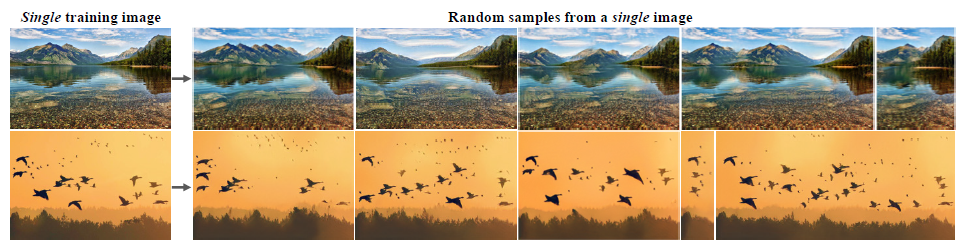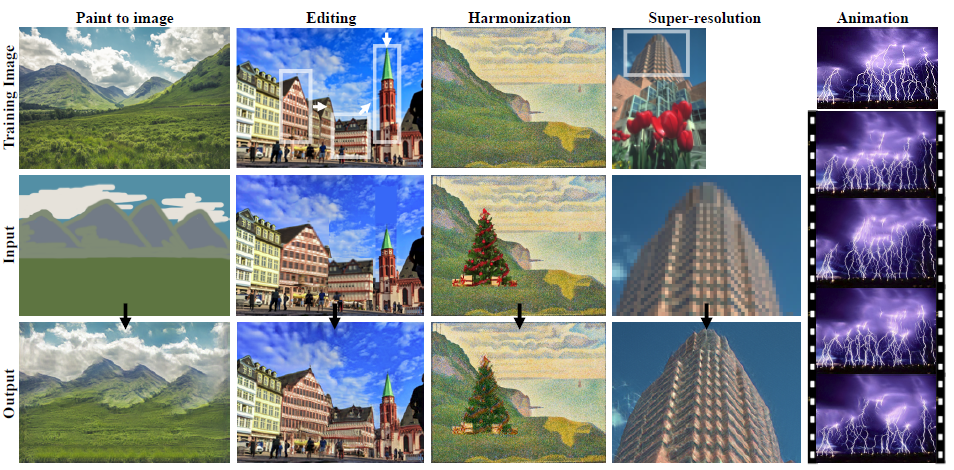### 网络结构• 由数组不同尺度大小的generator和discriminator组成. 从最小尺度的GAN开始训练, 训练完成后冻结, 继而转向下一个更大尺度的GAN. 小尺度GAN的输出会用做下一步更大尺度GAN的输入. 以此种方式, 来提取不同尺度大小的纹理信息. 同时, 由于GAN在生成纹理信息时, 需要的是小的感受域, 故卷积核和卷积层数不能过大过深, 该文的$G_N$只用了5层ConvBlocks. 这也是因为训练集只有单张图片, 为了防止过拟合而不能使用大的网络容量. $D_N$也是同样的5层ConvBlocks.

$G_N$是如下图所示的ResBlock形式.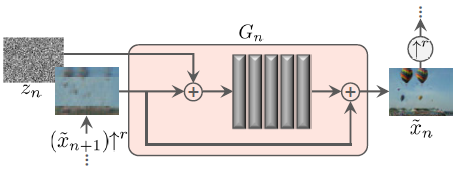### 损失函数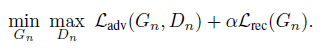• 因为之前没用过GAN, 所以对GAN loss这块不是很懂, 趁这个机会clone了一下sinGAN的源码, 把GAN loss的机理弄明白了.接下来分步记录一下.

loss向后传播的过程分两步, 第一步先更新$D_N$, 目标是使$D_N$在面对$X_{real}$时能判断概率是1, 在面对$X_{fake}$时能判断概率是0; 第二步再更新$G_N$, 目标是使生成的$X_{fake}$能使$D_N$错误地判断出概率是1.

1. 更新$D_N$
先搬出二进制交叉熵的公式:
$H(P \mid Q) = -( plogq + (1-p)log(1-q) )$
其中, $P$是Ground Truth属于正例的概率, 值为0或1; $Q$是prediction属于正例的概率, 值为0或1.
针对正样本, $P=1$, 此时的交叉熵为:
$-( 1 \ast logq + 0 \ast log(1-q) ) = -logq = -logD(X_{real})$
针对负样本, $P=0$, 此时的交叉熵为:
$-( 0 \ast logq + 1 \ast log(1-q) ) = -log(1-q) = -log(1-D(X_{fake}))$
综上, 要想使$D$达到上一段所说的目的, 需要让交叉熵最小, 即:
$loss_{D} = -logD(X_{real}) - log(1-D(X_{fake}))$
具体到代码, 只需要让loss有如下形式即可,
$loss_{D} = -D(X_{real}) + D(X_{fake})$
2. (为了缓解GAN在训练过程中不稳定等一些问题, 代码还采用了gradient penalty项加在step 1中的$loss_{D}后$, to be done. 关于WGAN_GP的原理讲解, 已更新在GAN Loss一文中.)
3. 更新$G_N$
$G_N$生成的一定是负样本, 所以有同上的交叉熵:
$-log(1-D(X_{fake}))$
要想让$G$达到上一段所说的目的, 需要让交叉熵最大, 即:
$loss_{G} = -(-log(1-D(X_{fake}))) = log(1-D(X_{fake}))$
具体到代码, 只需要有:
$loss_{G} = -D(X_{fake})$
4. Reconstruction loss 重构损失项被加在step3中的$loss_{G}$后. 文中对该项给出的解释是: The reconstruction loss Lrec insures the existence of a specific set of noise maps that can produce xn, an important feature for image manipulation, 我不是很理解, to be done.

### 模型效果

• 大部门是定性结果, 在部分任务(如超分辨率)上有定量的指标如下, 比较distortion(失真)和perceptual quality(感知质量)两个指标. 在单张训练集上实现了接近SOTA的分数.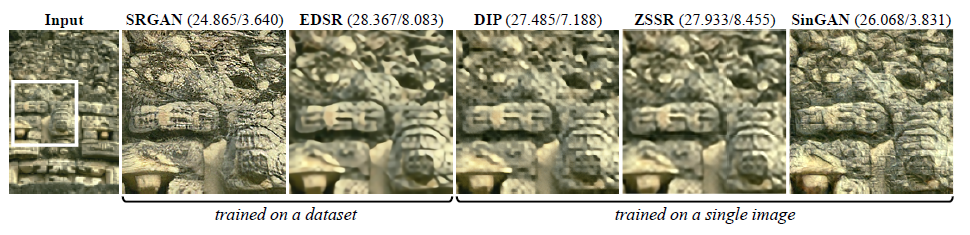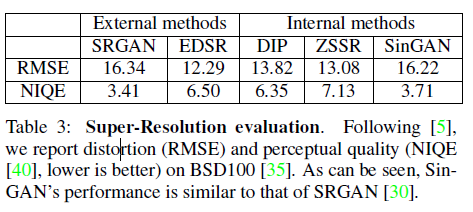### 局限性

• 该模型也存在适用性, 由于训练集只有一张图, 在语义多样性方面受到局限. 例如, 图片中只有一只狗时, 生成的图不会有许多种类不同的狗. 同时, 整体纹理不具有大范围相似性时, 效果也不是特别好. 例如, 自己拿一只小猫的图片训练后, 生成的图片只学习到了猫身上的斑纹特点, 并没有学习到猫的语义轮廓信息. 关于这一点, 作者自己也在文末加以了说明: Internal learning is inherently limited in terms of semantic diversity compared to externally trained generation methods.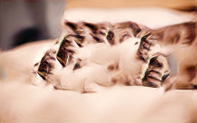##### 项目链接:

http://webee.technion.ac.il/people/tomermic/SinGAN/SinGAN.htm